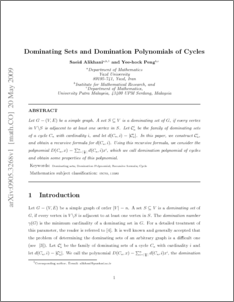# Dominating Sets and Domination Polynomials of Cycles

## Citation

Alikhania, Saeid and Yee-hock, Peng (2008) Dominating Sets and Domination Polynomials of Cycles. Global Journal of Pure And Applied Mathematics, 4 (2). pp. 202-210.

## Abstract

Let G = (V,E) be a simple graph. A set S ⊆ V is a dominating set of G, if every vertex in V \S is adjacent to at least one vertex in S. Let Ci n be the family of dominating sets of a cycle Cn with cardinality i, and let d(Cn, i) = |Ci n|. In this paper, we construct Ci n,and obtain a recursive formula for d(Cn, i). Using this recursive formula, we consider the polynomial D(Cn, x) = Pn i=⌈ n 3 ⌉ d(Cn, i)xi, which we call domination polynomial of cycles and obtain some properties of this polynomial.Preview
PDF
136.pdfView Item